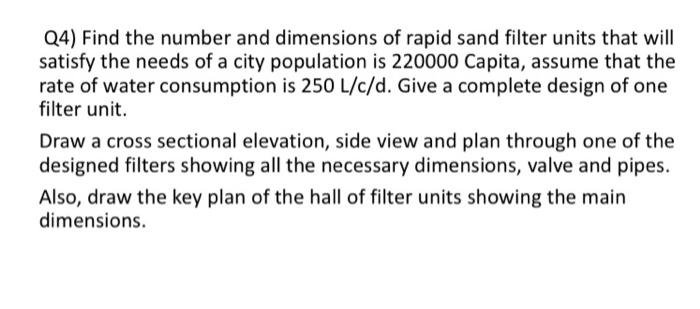Home / Expert Answers / Civil Engineering / q4-find-the-number-and-dimensions-of-rapid-sand-filter-units-that-will-satisfy-the-needs-of-a-city-pa714

# (Solved): Q4) Find the number and dimensions of rapid sand filter units that will satisfy the needs of a city ...Q4) Find the number and dimensions of rapid sand filter units that will satisfy the needs of a city population is 220000 Capita, assume that the rate of water consumption is $$250 \mathrm{~L} / \mathrm{c} / \mathrm{d}$$. Give a complete design of one filter unit. Draw a cross sectional elevation, side view and plan through one of the designed filters showing all the necessary dimensions, valve and pipes. Also, draw the key plan of the hall of filter units showing the main dimensions.

We have an Answer from Expert